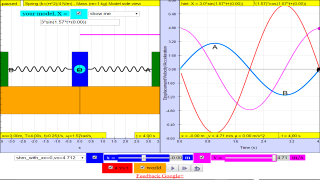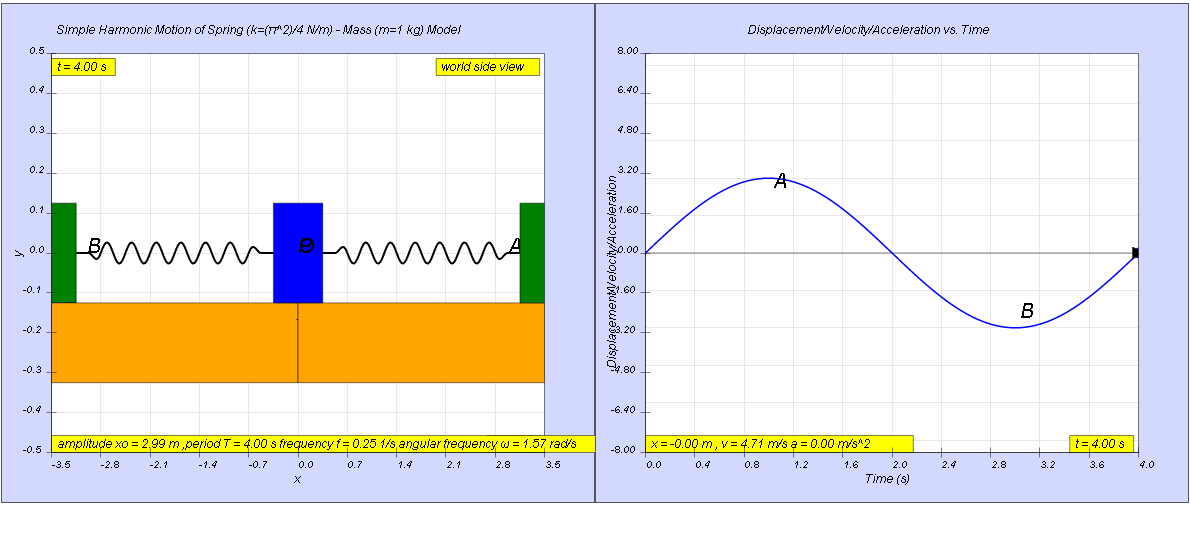SHM15

### 1.2.9 Example

A body undergoes a simple harmonic motion with amplitude 3.0 m and period 4.00 s. Find the

a)    angular frequency,
b)    maximum speed,
c)    maximum acceleration,
d)    acceleration of the body when it is 1.0 m from the equilibrium point, and
e)    speed of the body when it is 1.0 m from the equilibrium point.

[ 1.57 rad/s, 4.7 m/s , 7.4 m/s^2 , -2.5 m/s^2, 4.4 m/s ]

##### 1.2.9.1 Solution:

a) angular frequency , ω = $\genfrac{}{}{0.1ex}{}{2\pi }{T}=\genfrac{}{}{0.1ex}{}{2\pi }{4}=1.57\genfrac{}{}{0.1ex}{}{rad}{s}$

b) maximum speed, v0 = x0ω = (3.00)(1.57) = 0.047 m/s

c) maximum acceleration, |a0| = x0ω2 = (3.00)(1.57)2 = 7.4 m/s2

d) when x = 1.00, acceleration, a = -ω2x = (1.57)2(1.00) = -2.5 m/s2

e) when x = 1.00, speed, v = x0ω = (3.00)(1.57) = 4.4 m/s

### Translations

Code Language Translator Run### Software Requirements

SoftwareRequirements

 Android iOS Windows MacOS with best with Chrome Chrome Chrome Chrome support full-screen? Yes. Chrome/Opera No. Firefox/ Samsung Internet Not yet Yes Yes cannot work on some mobile browser that don't understand JavaScript such as..... cannot work on Internet Explorer 9 and below

### CreditsThis email address is being protected from spambots. You need JavaScript enabled to view it.

### end faq

http://iwant2study.org/lookangejss/02_newtonianmechanics_8oscillations/ejss_model_SHM15/SHM15_Simulation.xhtml